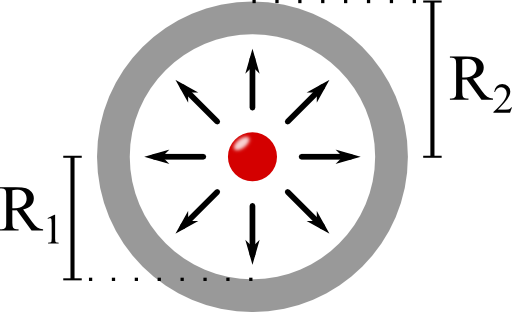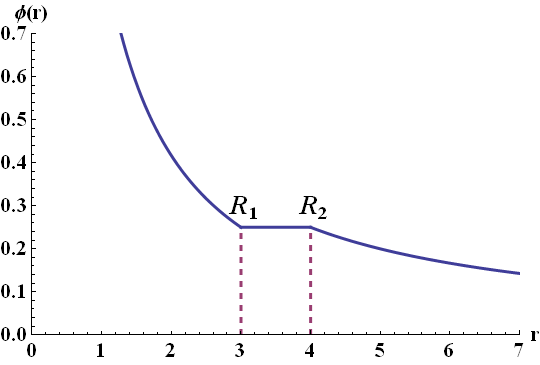## A Charge inside a Metallic ShellA charge close to a metal induces surface charges. But what happens if one puts some charge inside of a metallic shell? What will happen to the field in- and outside of this cavity? Also find out what how the shell screens the inner structure of an arbitrary charge distribution.## Problem Statement

Consider a point charge $$q$$ centered inside of an uncharged metallic shell. The shell is not grounded and has inner and outer radii $$R_{1}$$ and $$R_{2}$$.

Calculate the electrostatic potential $$\phi\left(\mathbf{r}\right)$$ and the surface charge densities $$\eta_{1/2}$$ at the inner and outer terminations of the shell.

Finally discuss what happens if an arbitrary charge distribution is put inside the shell. How could you measure anything else but the total charge?

## Hints

What does the notion "metal" imply for the electric field inside the shell?

Can you explain what should happen with the electrons inside the metallic shell if a charged body is placed inside the cavity?

## Show Solution

As in the problem The Electric Field of a Point Charge we have to apply Gauss's law to find the potential for the different regions of the problem. Using the boundary conditions and our model of a perfect metal we will be able to determine the charge distributions on the boundaries of the shell. At last, we will find a simple explanation for the screening of the shell.

## The Field Outside of the Shell

Let us first try to find the potential in the outer region. If we think of a surface containing the shell we will always find that the charge inside of this surface must be equal to $$q$$. Otherwise we would have included some additional charge! Also, there is no reason to assume that the potential is not rotationally symmetric. For such a situation we already know the solution - in the outer region it must be simply the field of a point charge $$q$$: $\begin{eqnarray*} \phi\left(\mathbf{r}\right) & = & \frac{1}{4\pi\epsilon_{0}}\frac{q}{r}\ \mathrm{for}\ r>R_{2}\ .\end{eqnarray*}$

In electrostatics a metal may be characterized as a material that does not allow any electric field inside of it - the free electrons will move until any external electric field is cancelled. So we know already that inside the shell the potential is constant (remember $$\mathbf{E}\left(\mathbf{r}\right)=-\nabla \phi\left(\mathbf{r}\right)$$). Since $$\phi$$ is continuous, we find $\begin{eqnarray*}\phi\left(\mathbf{r}\right) & = & \frac{1}{4\pi\epsilon_{0}}\frac{q}{R_{2}}\ \mathrm{for}\ r\in\left[R_{1},R_{2}\right]\ .\end{eqnarray*}$

## The Surface Charges - Gauss's Law

Now we have everything we need to calculate the surface charges. Remember again Gauss's law, $\begin{eqnarray*}\nabla\cdot\mathbf{E}\left(\mathbf{r}\right) & = & \frac{\rho\left(\mathbf{r}\right)}{\epsilon_{0}}\end{eqnarray*}$implies$\begin{eqnarray*}\int_{\partial V}\mathbf{E}\left(\mathbf{r}\right)\cdot\mathbf{n}dS & = & \frac{\int_{V}\rho\left(\mathbf{r}\right)dV}{\epsilon_{0}}\ .\end{eqnarray*}$

Because of the symmetry of the problem, the electric field has only a radial component $$E_{r}$$. If we compare the field at at the radii $$R_{2}\pm\delta$$ using a very small $$\delta$$ we can relate the total charge $$q_{2}$$ at $$R_{2}$$ to the electric field, see the figure on the left

Integration of the latter equation yields$\begin{eqnarray*} 4\pi R_{2}^{2}\left(E_{r}\left(r=R_{2}+\delta\right)-E_{r}\left(r=R_{2}-\delta\right)\right) & = & \frac{q_{2}}{\epsilon_{0}}\ .\end{eqnarray*}$Now, $\begin{eqnarray*} \lim_{\delta\rightarrow0}E_{r}\left(r=R_{2}+\delta\right) & = & -\partial_{r}\phi\left(R_{2}\right)\\ & = & \frac{1}{4\pi\epsilon_{0}}\frac{q}{R_{2}^{2}}\ . \end{eqnarray*}$Since furthermore $$E_{r}\left(r=R_{2}-\delta\right)=0$$ (inside the metallic shell!), we find $\begin{eqnarray*} q_{2} & = & q\ .\end{eqnarray*}$As we can see from the boundary condition in $$\mathbf{E}$$, a jump in the derivative of the potential always implies a surface charge.

It follows directly that the inner induced charge $\begin{eqnarray*} q_{1} & = & -q \end{eqnarray*}$since the sum of all charges, $$q+q_{1}+q_{2}$$, has to be $$q$$. The surface charge densities $$\eta_{1/2}$$ are then given by the ratio of the induced charge per surface, $\begin{eqnarray*} \eta_{1/2} & = & \frac{q_{1/2}}{4\pi R_{1/2}^{2}}\ . \end{eqnarray*}$

## Putting it All TogetherUsing the continuity of $$\phi$$ at $$r=R_{1}$$ finally yields the entire potential $\begin{eqnarray*}\phi\left(r\right) & = & \frac{q}{4\pi\epsilon_{0}}\begin{cases}\frac{1}{r}+\frac{1}{R_{2}}-\frac{1}{R_{1}} & r<R_{1}\\ \frac{1}{R_{2}} & r\in\left[R_{1},R_{2}\right]\\\frac{1}{r}\ , & r>R_{2} \end{cases}\ .\end{eqnarray*}$ On the right you can see a plot of this potential for a charge in a metallic shell (in arbitrary units).

You might say that this approach was too complicated. Of course we could have directly argued that the inner surface charge $$q_{1}$$ must be the negative of $$q$$. Otherwise, there would be an electric field inside the shell. Nevertheless we came to the same conclusion using some useful concepts. These may help us in other more complicated problems.

## The Screening of a Metallic Shell

Now to the last part of the problem: What happens if there was an arbitrary charge distribution inside of the cavity? In this case, the inner charge surface charge distribution $$\eta_1\left(\theta,\varphi\right)$$ might be a complicated function. Nevertheless, the outer charge distribution must be a constant! The reason is that the the metallic surface cannot have tangential electric field components. Otherwise charges would move along the surface until these components vanish. However, if the charge distribution is just a constant, the field outside of the shell will always look like a point charge, any other higher order moments (dipole, ...) just vanishes! This property is often called screening.